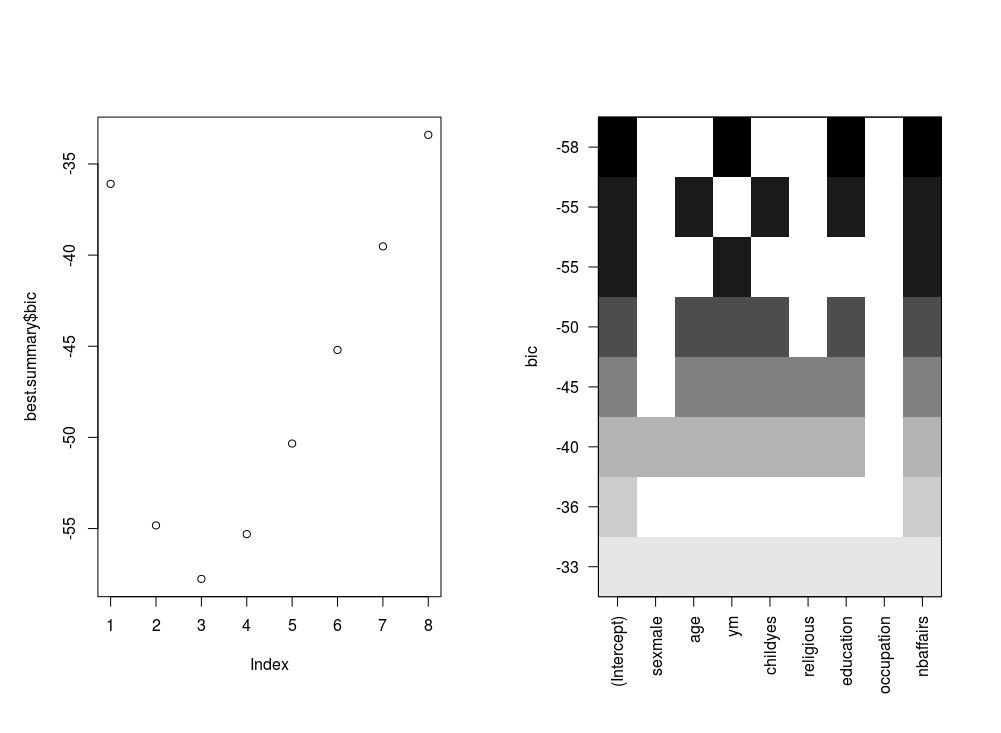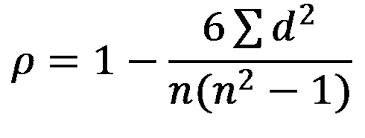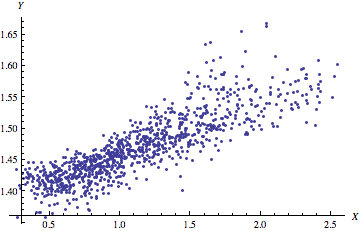# Best Subset Regression in R

In this post, we will take a look at best subset regression. Best subset regression fits a model for all possible feature or variable combinations and the decision for the most appropriate model is made by the analyst based on judgment or some statistical criteria.

Best subset regression is an alternative to both Forward and Backward stepwise regression. Forward stepwise selection adds one variable at a time based on the lowest residual sum of squares until no more variables continue to lower theresidual sum of squares. Backward stepwise regression starts with all variables in the model and removes variables one at a time. The concern with stepwise methods is they can produce biased regression coefficients, conflicting models, and inaccurate confidence intervals.

Best subset regression bypasses these weaknesses of stepwise models by creating all models possible and then allowing you to assess which variables should be included in your final model. The one drawback to best subset is that a large number of variables means a large number of potential models, which can make it difficult to make a decision among several choices.

In this post, we will use the “Fair” dataset from the “Ecdat” package to predict marital satisfaction based on age, Sex, the presence of children, years married, religiosity, education, occupation, and the number of affairs in the past year. Below is some initial code.

``library(leaps);library(Ecdat);library(car);library(lmtest)``
`data(Fair)`

We begin our analysis by building the initial model with all variables in it. Below is the code

``````fit<-lm(rate~.,Fair)
summary(fit)``````
``````##
## Call:
## lm(formula = rate ~ ., data = Fair)
##
## Residuals:
##     Min      1Q  Median      3Q     Max
## -3.2049 -0.6661  0.2298  0.7705  2.2292
##
## Coefficients:
##              Estimate Std. Error t value Pr(>|t|)
## (Intercept)  3.522875   0.358793   9.819  < 2e-16 ***
## sexmale     -0.062281   0.099952  -0.623  0.53346
## age         -0.009683   0.007548  -1.283  0.20005
## ym          -0.019978   0.013887  -1.439  0.15079
## childyes    -0.206976   0.116227  -1.781  0.07546 .
## religious    0.042142   0.037705   1.118  0.26416
## education    0.068874   0.021153   3.256  0.00119 **
## occupation  -0.015606   0.029602  -0.527  0.59825
## nbaffairs   -0.078812   0.013286  -5.932 5.09e-09 ***
## ---
## Signif. codes:  0 '***' 0.001 '**' 0.01 '*' 0.05 '.' 0.1 ' ' 1
##
## Residual standard error: 1.03 on 592 degrees of freedom
## Multiple R-squared:  0.1405, Adjusted R-squared:  0.1289
## F-statistic:  12.1 on 8 and 592 DF,  p-value: 4.487e-16``````

The initial results are already interesting even though the r-square is low. When couples have children the have less marital satisfaction than couples without children when controlling for the other factors and this is the strongest regression weight. In addition, the more education a person has there is an increase in marital satisfaction. Lastly, as the number of affairs increases there is also a decrease in marital satisfaction. Keep in mind that the “rate” variable goes from 1 to 5 with one meaning a terrible marriage to five being a great one. The mean marital satisfaction was 3.52 when controlling for the other variables.

We will now create our subset models. Below is the code.

``````sub.fit<-regsubsets(rate~.,Fair)
best.summary<-summary(sub.fit)``````

In the code above we create the sub models using the “regsubsets” function from the “leaps” package and saved it in the variable called “sub.fit”. We then saved the summary of “sub.fit” in the variable “best.summary”. We will use the “best.summary” “sub.fit variables several times to determine which model to use.

There are many different ways to assess the model. We will use the following statistical methods that come with the results from the “regsubset” function.

• Mallow’ Cp
• Bayesian Information Criteria

We will make two charts for each of the criteria above. The plot to the left will explain how many features to include in the model. The plot to the right will tell you which variables to include. It is important to note that for both of these methods, the lower the score the better the model. Below is the code for Mallow’s Cp.

``````par(mfrow=c(1,2))
plot(best.summary\$cp)
plot(sub.fit,scale = "Cp")``````The plot on the left suggests that a four feature model is the most appropriate. However, this chart does not tell me which four features. The chart on the right is read in reverse order. The high numbers are at the bottom and the low numbers are at the top when looking at the y-axis. Knowing this, we can conclude that the most appropriate variables to include in the model are age, children presence, education, and number of affairs. Below are the results using the Bayesian Information Criterion

``````par(mfrow=c(1,2))
plot(best.summary\$bic)
plot(sub.fit,scale = "bic")``````These results indicate that a three feature model is appropriate. The variables or features are years married, education, and number of affairs. Presence of children was not considered beneficial. Since our original model and Mallow’s Cp indicated that presence of children was significant we will include it for now.

Below is the code for the model based on the subset regression.

``````fit2<-lm(rate~age+child+education+nbaffairs,Fair)
summary(fit2)``````
``````##
## Call:
## lm(formula = rate ~ age + child + education + nbaffairs, data = Fair)
##
## Residuals:
##     Min      1Q  Median      3Q     Max
## -3.2172 -0.7256  0.1675  0.7856  2.2713
##
## Coefficients:
##              Estimate Std. Error t value Pr(>|t|)
## (Intercept)  3.861154   0.307280  12.566  < 2e-16 ***
## age         -0.017440   0.005057  -3.449 0.000603 ***
## childyes    -0.261398   0.103155  -2.534 0.011531 *
## education    0.058637   0.017697   3.313 0.000978 ***
## nbaffairs   -0.084973   0.012830  -6.623 7.87e-11 ***
## ---
## Signif. codes:  0 '***' 0.001 '**' 0.01 '*' 0.05 '.' 0.1 ' ' 1
##
## Residual standard error: 1.029 on 596 degrees of freedom
## Multiple R-squared:  0.1352, Adjusted R-squared:  0.1294
## F-statistic: 23.29 on 4 and 596 DF,  p-value: < 2.2e-16``````

The results look ok. The older a person is the less satisfied they are with their marriage. If children are present the marriage is less satisfying. The more educated the more satisfied they are. Lastly, the higher the number of affairs indicate less marital satisfaction. However, before we get excited we need to check for collinearity and homoscedasticity. Below is the code

``vif(fit2)``
``````##       age     child education nbaffairs
##  1.249430  1.228733  1.023722  1.014338``````

No issues with collinearity.For vif values above 5 or 10 indicate a problem. Let’s check for homoscedasticity

``````par(mfrow=c(2,2))
plot(fit2)``````The normal qqplot and residuals vs leverage plot can be used for locating outliers. The residual vs fitted and the scale-location plot do not look good as there appears to be a pattern in the dispersion which indicates homoscedasticity. To confirm this we will use Breusch-Pagan test from the “lmtest” package. Below is the code

``bptest(fit2)``
``````##
##  studentized Breusch-Pagan test
##
## data:  fit2
## BP = 16.238, df = 4, p-value = 0.002716``````

There you have it. Our model violates the assumption of homoscedasticity. However, this model was developed for demonstration purpose to provide an example of subset regression.# Spearman Rank Correlation

Spearman rank correlation aka ρ is used to measure the strength of the relationship between two variables. You may be already wondering what is the difference between Spearman rank correlation and Person product moment correlation. The difference is that Spearman rank correlation is a non-parametric test while Person product moment correlation is a parametric test.

A non-parametric test does not have to comply with the assumptions ofparametric test such as the data being normally distributed. This allows a researcher to still make inferences from data that may not have normality. In addition, non-parametric test are used for data that is at the ordinal or nominal level. In many ways, Spearman correlation and Pearson product moment correlation compliment each other. One is used in non-parametric statistics and the other for parametric statistics and each analyzes the relationship between variables.

If you get suspicious results from your Pearson product moment correlation analysis or your data lacks normality Spearman rank correlation may be useful for you if you still want to determine if there is a relationship between the variables. Spearmen correlation works by ranking the data within each variable. Next, the Pearson product moment correlation is calculated between the two sets of rank variables. Below are the assumptions of Spearman correlation test.

• Subjects are randomly selected
• Observations are at the ordinal level at least

Below are the steps of Spearman correlation

1. Setup the hypotheses
1. H0: There is no correlation between the variables
2. H1: There is a correlation between the variables
2. Set the level of significance
3. Calculate the degrees of freedom and find the t-critical value (computer does this for you)
4. Calculate the value of Spearman correlation or ρ (computer does this for you)
5. Calculate the t-value(computer does this for you) and make a statistical decision
6. State conclusion

Here is an example

A clerk wants to see if there is a correlation between the overall grade students get on an exam and  the number of words they wrote for their essay. Below are the results

1                             79                           147
2                             76                           143
3                             78                           147
4                             84                           168
5                             90                           206
6                             83                           155
7                             93                           192
8                             94                           211
9                             97                           209
10                           85                           187
11                           88                           200
12                           82                           150

Note: The computer will rank the data of each variable with a rank of 1 being the highest value of a variable and a rank 12 being the lowest value of a variable. Remember that the computer does this for you.

Step 1: State hypotheses
H0: There is no relationship between grades and words on the essay
H1: There is a relationship between grades and words on the essay

Step 2: Determine level of significance
Level set to 0.05

Step 3: Determine critical t-value
t = + 2.228 (computer does this for you)

Step 4: Compute Spearman correlation
ρ = 0.97 (computer does this for you)
Note: This correlation is very strong. Remember the strongest relationship possible is + 1

Step 5: Calculate t-value and make a decision
t = 12.62   ( the computer does this for you)
Since the computed t-value of 12.62 is greater than the t-critical value of 2.228 we reject the null hypothesis

Step 6: Conclusion
Since the null hypotheses are rejected, we can conclude that there is evidence that there is a strong relationship between exam grade and the number of words written on an essay. This means that a teacher could tell students they should write longer essays if they want a higher grade on exams# Correlation

A correlation is a statistical method used to determine if a relationship exists between variables.  If there is a relationship between the variables itindicates a departure from independence. In other words, the higher the correlation the stronger the relationship and thus the more the variables have in common at least on the surface.

There are four common types of relationships between variables there are the following

1. positive-Both variables increase or decrease in value
2. Negative- One variable decreases in value while another increases.
3. Non-linear-Both variables move together for a time then one decreases while the other continues to increase
4. Zero-No relationship

The most common way to measure the correlation between variables is the Pearson product-moment correlation aka correlation coefficient aka r.  Correlations are usually measured on a standardized scale that ranges from -1 to +1. The value of the number, whether positive or negative, indicates the strength of the relationship.

The Person Product Moment Correlation test confirms if the r is statistically significant or if such a relationship would exist in the population and not just the sample. Below are the assumptions

• Subjects are randomly selected
• Both populations are normally distributed

Here is the process for finding the r.

1. Determine hypotheses
• H0: = 0 (There is no relationship between the variables in the population)
• H0: r ≠ 0 (There is a relationship between the variables in the population)
2. Decided what the level of significance will be
3. Calculate degrees of freedom to determine the t critical value (computer does this)
4. Calculate Pearson’s (computer does this)
5. Calculate t value (computer does this)
6. State conclusion.

Below is an example

A clerk wants to see if there is a correlation between the overall grade students get on an exam and the number of words they wrote for their essay. Below are the results

1                             79                           147
2                             76                           143
3                             78                           147
4                             84                           168
5                             90                           206
6                             83                           155
7                             93                           192
8                             94                           211
9                             97                           209
10                          85                           187
11                          88                           200
12                          82                           150

Step 1: State Hypotheses
H0: There is no relationship between grade and the number of words on the essay
H1: There is a relationship between grade and the number of words on the essay

Step 2: Level of significance
Set to 0.05

Step 3: Determine degrees of freedom and t critical value
t-critical = + 2.228 (This info is found in a chart in the back of most stat books)

Step 4: Compute r
r = 0.93                       (calculated by the computer)

Step 5: Decision rule. Calculate t-value for the r

t-value for r = 8.00  (Computer found this)

Since the computed t-value of 8.00 is greater than the t-critical value of 2.228 we reject the null hypothesis.

Step 6: Conclusion
Since the null hypothesis was rejected, we conclude that there is evidence that a strong relationship between the overall grade on the exam and the number of words written for the essay. To make this practical, the teacher could tell the students to write longer essays if they want a better score on the test.

IMPORTANT NOTE

When a null hypothesis is rejected there are several possible relationships between the variables.

• Direct cause and effect
• The relationship between X and Y may be due to the influence of a third variable not in the model
• This could be a chance relationship. For example, foot size and vocabulary. Older people have bigger feet and also a larger vocabulary. Thus it is a nonsense relationship